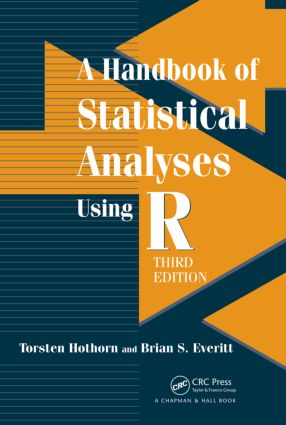# A Handbook of Statistical Analyses using R

## 3rd Edition

Chapman and Hall/CRC

##### Purchasing Options:\$ = USD
Paperback: 9781482204582
pub: 2014-06-25
\$72.95
x
Hardback: 9781138469792
pub: 2017-12-18
\$205.00
x
eBook (VitalSource) : 9780429169465
pub: 2014-05-30
from \$35.48

FREE Standard Shipping!

### Description

Like the best-selling first two editions, A Handbook of Statistical Analyses using R, Third Edition provides an up-to-date guide to data analysis using the R system for statistical computing. The book explains how to conduct a range of statistical analyses, from simple inference to recursive partitioning to cluster analysis.

New to the Third Edition

• Three new chapters on quantile regression, missing values, and Bayesian inference
• Extra material in the logistic regression chapter that describes a regression model for ordered categorical response variables
• More detailed explanations of R code
• New section in each chapter summarizing the results of the analyses
• Updated version of the HSAUR package (HSAUR3), which includes some slides that can be used in introductory statistics courses

Whether you’re a data analyst, scientist, or student, this handbook shows you how to easily use R to effectively evaluate your data. With numerous real-world examples, it emphasizes the practical application and interpretation of results.

### Reviews

“I truly appreciate how grounded in practicality this book is—and the way its chapters are structured really underlines this. Furthermore, all the datasets are interesting and vary widely in subject matter. If nothing else, this book is an excellent source of examples one might use to illustrate a variety of statistical techniques. … it offers a lot of good places to start if one wants to analyze data. … The book comes hand-in-hand with an R package, HSAUR3, with all the data and the code used in the text. The book is thus fully reproducible. Overall, it provides a great way for a statistician to get started doing a wide variety of things in the R environment. It would be particularly useful, then, for working statisticians looking to change their software. The book cites all the relevant packages one might need, which is quite nice for those attempting to navigate the vast array of packages freely available, and is quite clear in its presentation of the code. Between this and the datasets, it makes for quite a valuable and enjoyable reference.”

The American Statistician, August 2015

"… a handy primer for using R to perform standard statistical data analysis. … students, analysts, professors, and scientists: if you are looking to add R to your toolkit for analyzing data statistically, then this book will get you there."

—Kendall Giles on his blog, September 2014

Praise for the Second Edition:

"I find the book by Everitt and Hothorn quite pleasant and bound to fit its purpose. The layout and presentation [are] nice. It should appeal to all readers as it contains a wealth of information about the use of R for statistical analysis. Included seasoned R users: When reading the first chapters, I found myself scribbling small lightbulbs in the margin to point out features of R I was not aware of. In addition, the book is quite handy for a crash introduction to statistics for (well-enough motivated) nonstatisticians."

International Statistical Review (2011), 79

"… an extensive selection of real data analyzed with [R] … Viewed as a collection of worked examples, this book has much to recommend it. Each chapter addresses a specific technique. … the examples provide a wide variety of partial analyses and the datasets cover a diversity of fields of study. … This handbook is unusually free of the sort of errors spell checkers do not find."

MAA Reviews, April 2011

Introduction

Density Estimation

Analysis Using R

Summary of Findings

Recursive Partitioning

Introduction

Recursive Partitioning

Analysis Using R

Summary of Findings

Scatterplot Smoothers and Additive Models

Introduction

Scatterplot Smoothers and Generalised Additive Models

Analysis Using R

Summary of Findings

Survival Analysis

Introduction

Survival Analysis

Analysis Using R

Summary of Findings

Quantile Regression

Introduction

Quantile Regression

Analysis Using R

Summary of Findings

Analysing Longitudinal Data I

Introduction

Analysing Longitudinal Data

Linear Mixed Effects Models

Analysis Using R

Prediction of Random Effects

The Problem of Dropouts

Summary of Findings

Analysing Longitudinal Data II

Introduction

Methods for Non-Normal Distributions

Analysis Using R: GEE

Analysis Using R: Random Effects

Summary of Findings

Simultaneous Inference and Multiple Comparisons

Introduction

Simultaneous Inference and Multiple Comparisons

Analysis Using R

Summary of Findings

Missing Values

Introduction

The Problems of Missing Data

Dealing with Missing Values

Imputing Missing Values

Analyzing Multiply Imputed Data

Analysis Using R

Summary of Findings

Meta-Analysis

Introduction

Systematic Reviews and Meta-Analysis

Statistics of Meta-Analysis

Analysis Using R

Meta-Regression

Publication Bias

Summary of Findings

Bayesian Inference

Introduction

Bayesian Inference

Analysis Using R

Summary of Findings

Principal Component Analysis

Introduction

Principal Component Analysis

Analysis Using R

Summary of Findings

Multidimensional Scaling

Introduction

Multidimensional Scaling

Analysis Using R

Summary of Findings

Cluster Analysis

Introduction

Cluster Analysis

Analysis Using R

Summary of Findings